# V2/2 u (d) Using the identity V2 – (V2 sin 0)2 = V2cos 0 when cos...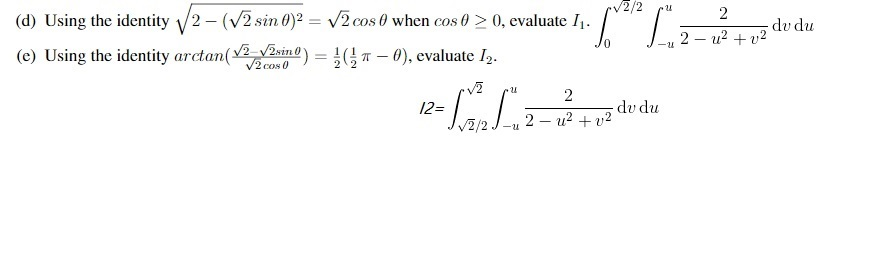V2/2 u (d) Using the identity V2 – (V2 sin 0)2 = V2cos 0 when cos 0 > 0, evaluate I1. (e) Using the identity arctan( v22isine) = (1 - 0), evaluate 12. 2 du du 2 - 42 +02 0 2 du du 2 - 42 +02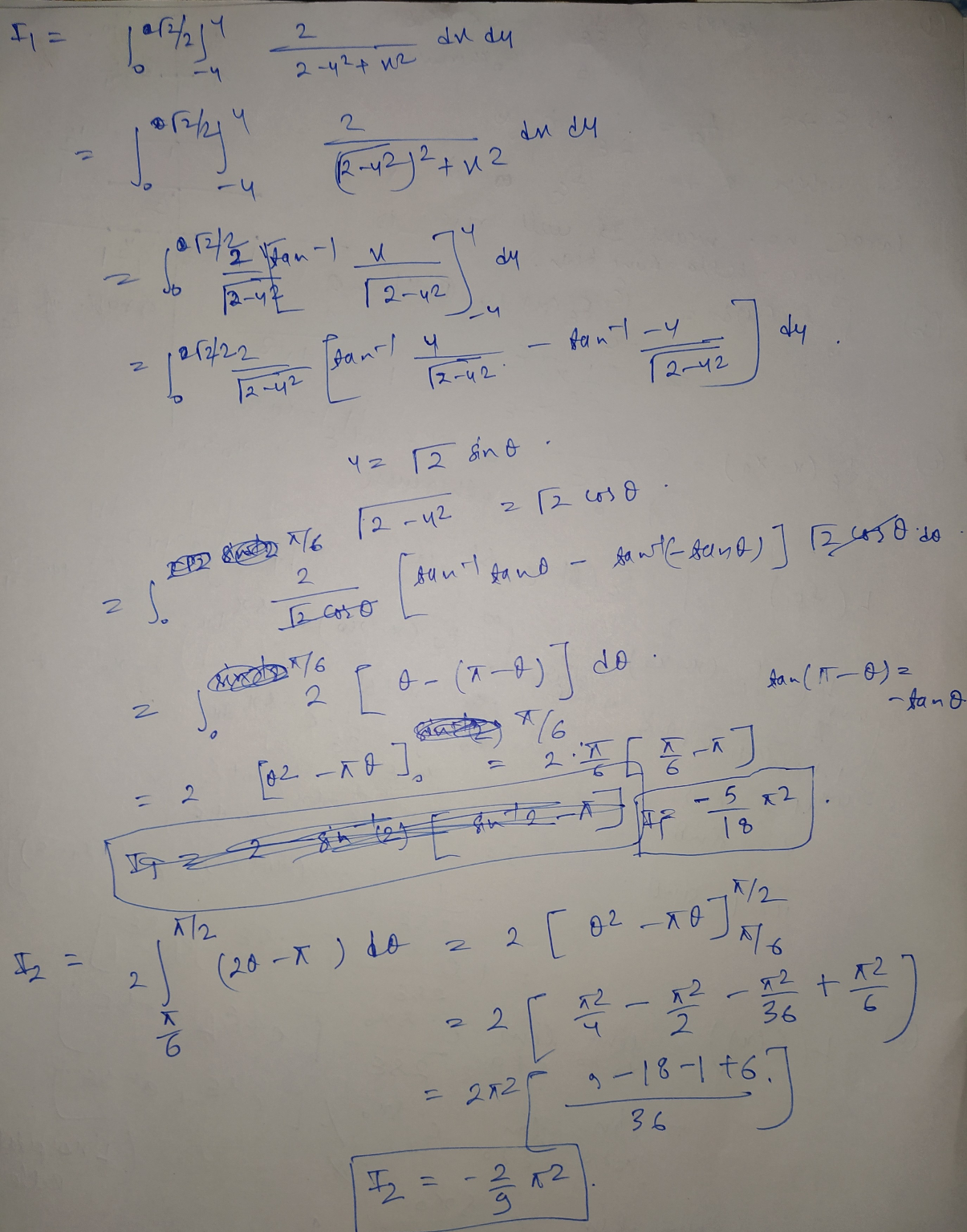#### Earn Coin

Coins can be redeemed for fabulous gifts.

Similar Homework Help Questions
• ###  sin 2B given sec B - 3 cos 2B and & sin >0. In what...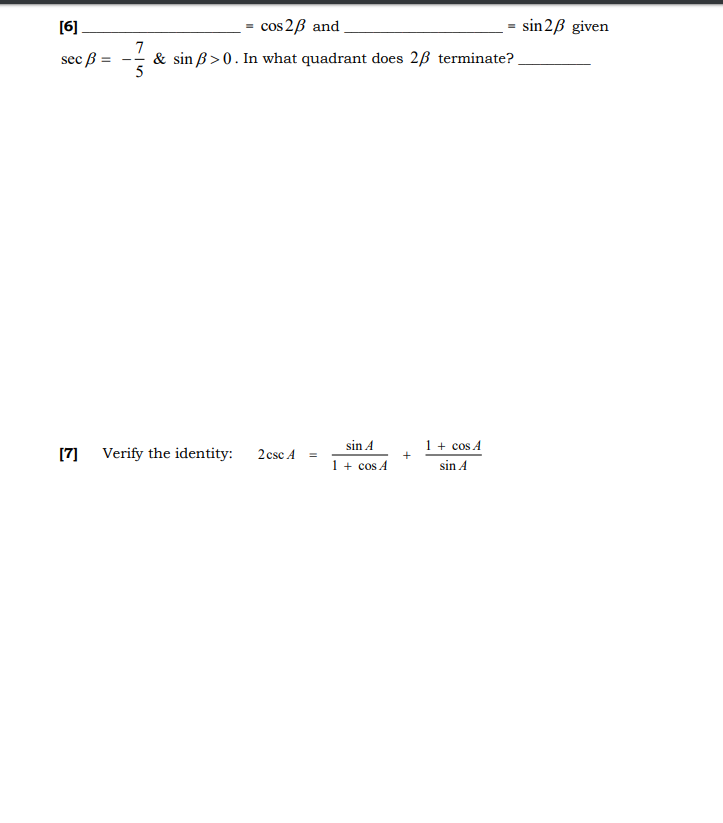sin 2B given sec B - 3 cos 2B and & sin >0. In what quadrant does 2B terminate? 7 5  Verify the identity: 2 csc A sin A 1 + cos A + 1 + cos A sin A

• ### State the quadrant in which lies. sin(8) <0, cos(8) < 0 OII III OIV 8 If...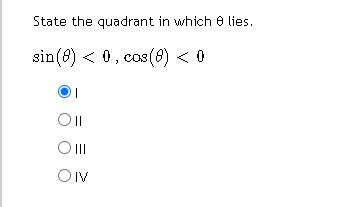State the quadrant in which lies. sin(8) <0, cos(8) < 0 OII III OIV 8 If sin() and 8 is in the 1st quadrant, find the exact value for cos(8). 9 cos(8) - > Next Question State the quadrant in which lies. tan(8) > 0, csc(8) < 0 01 OII O III OIV

• ### Using the identity sin? 0 + cos² 0 = 1, find the value of tan 6,...Using the identity sin? 0 + cos² 0 = 1, find the value of tan 6, to the nearest hundredth, if sin 0 = -0.62 and 3 < 0 < 27.

• ### Evaluate the following integral using residues: cos(bx)-cos(ax) I = dx. x2 Let a and b: real...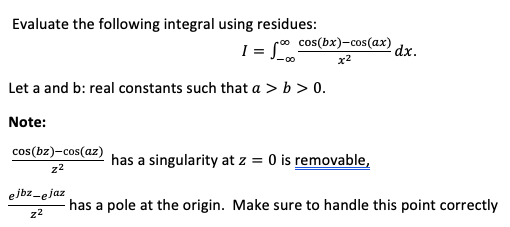Evaluate the following integral using residues: cos(bx)-cos(ax) I = dx. x2 Let a and b: real constants such that a > b >0. Note: cos(bz)-cos(az) has a singularity at z = 0 is removable, z2 ejbz-ejaz has a pole at the origin. Make sure to handle this point correctly 22

• ### Use induction and Pascal's identity to prove that (7) = 2" where n > 0.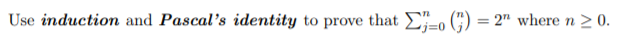Use induction and Pascal's identity to prove that (7) = 2" where n > 0.

• ### T Find the length of the curve e' cos(t) e' sin(t) for 0 < t <...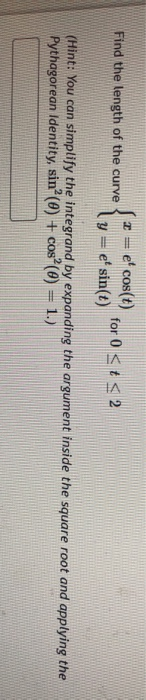T Find the length of the curve e' cos(t) e' sin(t) for 0 < t < 2 y (Hint: You can simplify the integrand by expanding the argument inside the square root and applying the Pythagorean identity, sinº (0) + cos²O) = 1.)

• ### Establish the identity 1 - cos 0 sin 0 + sin 0 1 - cos 0...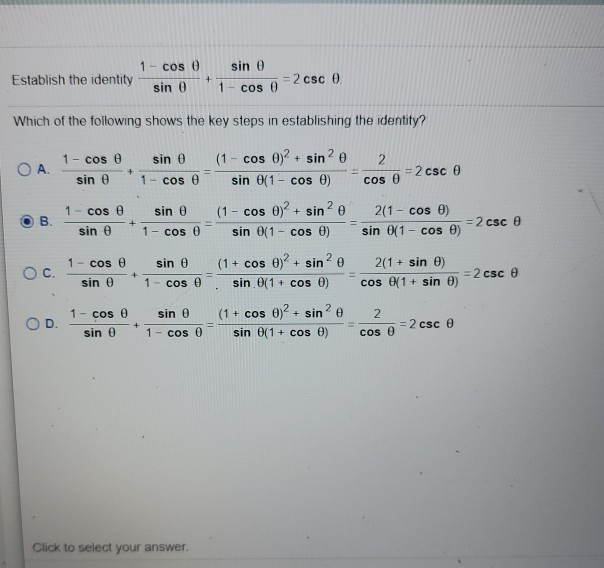Establish the identity 1 - cos 0 sin 0 + sin 0 1 - cos 0 = 2 csc 0. Which of the following shows the key steps in establishing the identity? 1 - cos e sin 0 ОА. + sin e 1 cos e 1 - cos e B + sin e 1 - cos 0 sin e (1 - cos 0)2 + sine 2 = 2 csc 6 sin 0(1 - cos ) cOS (1 - cos 02...

• ### Write the expression as an algebraic (nontrigonometric) expression in u, u> 0. cos (arctanu) cos (arctan...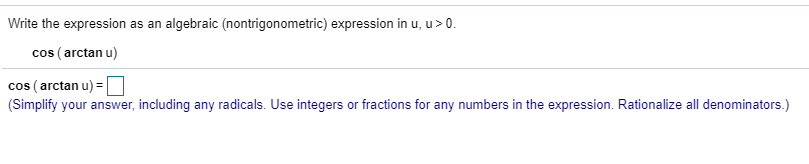Write the expression as an algebraic (nontrigonometric) expression in u, u> 0. cos (arctanu) cos (arctan u) = 0 (Simplify your answer, including any radicals. Use integers or fractions for any numbers in the expression. Rationalize all denominators.) The following function approximates the average monthly temperature y (in °F) in a city. Here x represents the month, where x= 1 corresponds to January, x=2 corresponds to February, and so on. Complete parts (a) (b). flx) = 11 sin [«- 49]+50...

• ### Evaluate the following integral using residues: I = { cos(bx)-cos(ax) dx. x2 Let a and b:...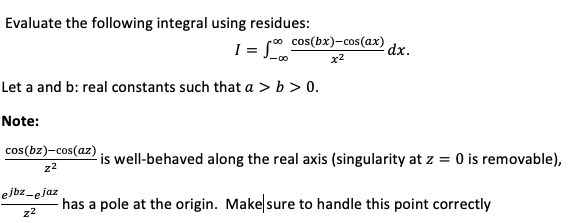Evaluate the following integral using residues: I = { cos(bx)-cos(ax) dx. x2 Let a and b: real constants such that a > b>0. Note: cos(bz)-cos(az) is well-behaved along the real axis (singularity at z = 0 is removable), ejbz-ejaz has a pole at the origin. Make sure to handle this point correctly 22

• ### Use a trigonometric identity to find exactly all solutions: cos 20 = sin , 0<o<21. Enter...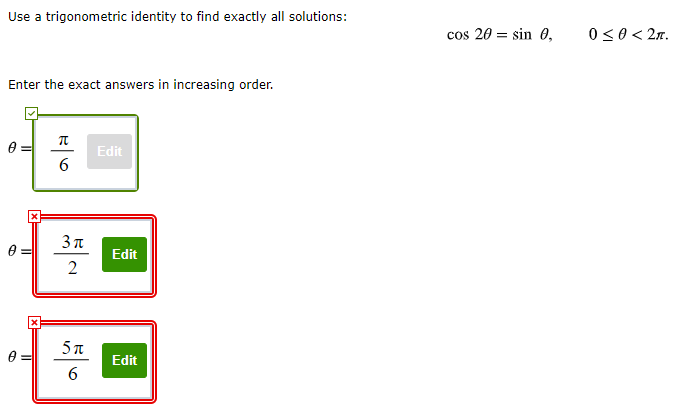Use a trigonometric identity to find exactly all solutions: cos 20 = sin , 0<o<21. Enter the exact answers in increasing order. O= Edit 6 31 Edit 2 II 5a 6 Edit

Free Homework App